## petgraph review: internals¶

petgraph is a rust library which allows you to work with graph data structures in rust.

In :
:dep petgraph = "0.4.13"
:dep petgraph-evcxr = "*"


## Internal representation of graphs by petgraph¶

This is the second part on a series about working with graphs in rust. The first part can be found here.

Petgraph supports four different internal representations for graphs. The most common one is Graph which I used throughout the first part of the tutorial.

## The Graph data structure¶

Graphs are stored like this:

pub struct Graph<N, E, Ty = Directed, Ix = DefaultIx> {
nodes: Vec<Node<N, Ix>>,
edges: Vec<Edge<E, Ix>>,
ty: PhantomData<Ty>,
}


...

pub struct Node<N, Ix = DefaultIx> {
/// Associated node data.
pub weight: N,
/// Next edge in outgoing and incoming edge lists.
next: [EdgeIndex<Ix>; 2],
}


...

pub struct Edge<E, Ix = DefaultIx> {
/// Associated edge data.
pub weight: E,
/// Next edge in outgoing and incoming edge lists.
next: [EdgeIndex<Ix>; 2],
/// Start and End node index
node: [NodeIndex<Ix>; 2],
}


...

My first impression when looking at this code was to be confused about the order of the 'outgoing and incoming edge list' so I looked in the source code and found that there is actually an enum which indexes the duple. It can be found in src/lib.rs.

pub enum Direction {
/// An Outgoing edge is an outward edge *from* the current node.
Outgoing = 0,
/// An Incoming edge is an inbound edge *to* the current node.
Incoming = 1
}


Basically, what is going on, is that each Node has two doubly linked lists of Edges associated with it. Now my next question, is how does a Directed Graph differ from an Undirected one in terms of internal representation?

I could have read the source code to figure that out, but I decided to have fun and instead visualize the datastructure by converting it to a graph.

In :
/*
In order to visualize the internals we need to make a bunch of fields public.
We do so via an unsafe cast, refered to as a transumtation in rust.
This method is described here:
https://users.rust-lang.org/t/is-private-really-private/7826/15
*/
extern crate petgraph;
use petgraph::*;
use petgraph::dot::Dot;
use petgraph::graph::*;
use petgraph::prelude::*;
use petgraph::data::*;
use std::marker::PhantomData;
use petgraph_evcxr::draw_graph;

pub struct PubNode<N, Ix> {
pub weight: N,
pub next: [EdgeIndex<Ix>; 2],
}

pub struct PubEdge<E, Ix = u64> {
/// Associated edge data.
pub weight: E,
/// Next edge in outgoing and incoming edge lists.
pub next: [EdgeIndex<Ix>; 2],
/// Start and End node index
pub node: [NodeIndex<Ix>; 2],
}

pub struct PubGraph<N, E, Ty = Directed, Ix = u64> {
pub nodes: Vec<PubNode<N, Ix>>,
pub edges: Vec<PubEdge<E, Ix>>,
pub ty: PhantomData<Ty>,
}

fn visualize_graph_internals<N, E, Ty, Ix>(g: &Graph<N, E, Ty, Ix>) -> Graph<String, String, petgraph::Directed>
where
N: std::fmt::Debug + Clone,
E: std::fmt::Debug + Clone,
Ty: EdgeType + Clone,
Ix: std::fmt::Debug + IndexType + Clone,
{
let mut v: Graph<String, String, petgraph::Directed> = Graph::new();
let mut _g: Graph<N, E, Ty, Ix> = g.clone();
let pub_g: PubGraph<N, E, Ty, Ix> = unsafe { std::mem::transmute(_g) };
let mut nodes: Vec<NodeIndex> = vec![NodeIndex::new(0); g.node_count()];
let mut edges: Vec<NodeIndex> = vec![NodeIndex::new(0); g.node_count()];
for node_id in g.node_indices() {
let w = format!("Node: {:?}", g.node_weight(node_id).unwrap());
}
for edge_id in g.edge_indices() {
let w = format!("Edge: {:?}", g.edge_weight(edge_id).unwrap());
}
let mut add_link = |start: NodeIndex, end: usize, collection: Vec<NodeIndex>, label: &str| {
match collection.get(end) {
Some(dest) => {
},
None => {
},
}
};
for (edge, edge_id) in pub_g.edges.iter().zip(g.edge_indices()) {
let vedge_id = edges[edge_id.index()];
}
for (node, node_id) in pub_g.nodes.iter().zip(g.node_indices()) {
let vnode_id = nodes[node_id.index()];
}
v
}


First lets look at an undirected graph; the list example from the previous article.

In :
let mut list : Graph<&str, &str, petgraph::Undirected> = Graph::new_undirected();
draw_graph(&list);

Out: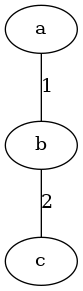In :
draw_graph(&visualize_graph_internals(&list));

Out: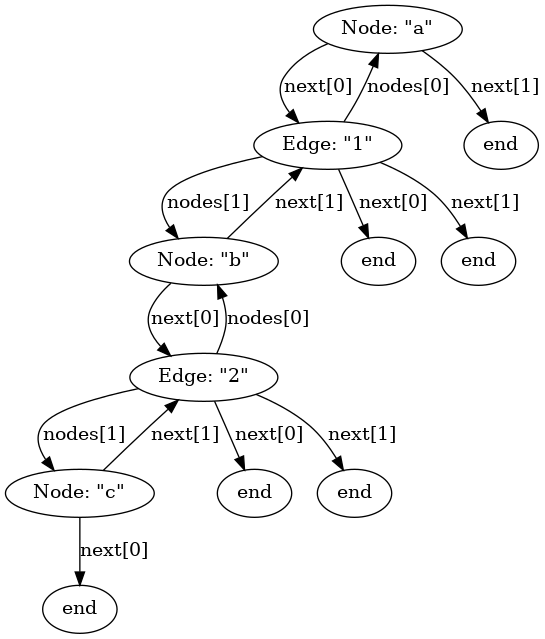Now lets look at a directed graph. I'll use the tree example.

In :
let mut tree : Graph<&str, &str, petgraph::Directed> = Graph::new();
draw_graph(&tree);

Out: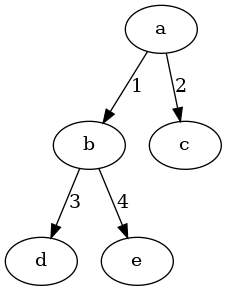In :
draw_graph(&visualize_graph_internals(&tree));

Out: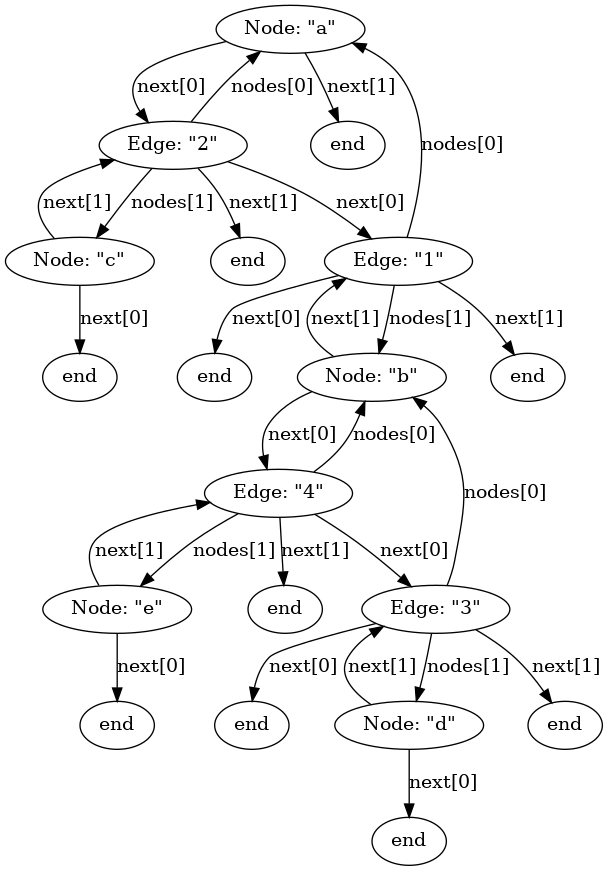As you can see, directed and undirected graphs have the exact same internal representation.

To answer a question from earier "how do we convert from a directed to an undirected graph?" We can do so "unsafely" simply by using transmute.

In :
let undirected_tree : Graph<&str, &str, petgraph::Undirected> = unsafe {std::mem::transmute(tree.clone())};
draw_graph(&undirected_tree);

Out: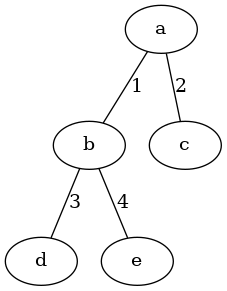Of course, since we did a transmute and it worked, we know that the representation should be the same, lets just verify that.

In :
draw_graph(&visualize_graph_internals(&undirected_tree));

Out:I'd like to point out some interesting consiquences of this data structure choice. One is that the doubly linked list of edges means that some nodes are "farther" from eachother than others in the data structure even though their theoretical distance is the same.

The distances between A → B and A → C are both 1 in the origional tree, but in the data structure, the distance from A → B is greater than that of A → C:

In :
use std::collections::HashMap;
use petgraph::algo::*;

let udt_internals = visualize_graph_internals(&undirected_tree);
{
fn print_path(
g: &Graph<String, String, Directed>,
start: &str,
end: &str,
){
let mut nodes: HashMap<&str, NodeIndex> = HashMap::new();
for i in g.node_indices() {
nodes.insert(g.node_weight(i).unwrap(), i);
}
let atob = astar(
g,
*nodes.get(start).unwrap(),
| id | { *nodes.get(end).unwrap() == id },
| _ | 0,
| _ | 0,
);
let atobv = atob.unwrap().1;
let atob_human: Vec<String> = atobv
.iter()
.map(| i | g.node_weight(*i).unwrap().clone())
.collect();
println!("Path from [{}] → [{}]:\n\t[{}]", start, end, atob_human.join("] → ["));
}
print_path(&udt_internals, "Node: \"a\"", "Node: \"b\"");
print_path(&udt_internals, "Node: \"a\"", "Node: \"c\"");
}

Path from [Node: "a"] → [Node: "b"]:
[Node: "a"] → [Edge: "2"] → [Edge: "1"] → [Node: "b"]
Path from [Node: "a"] → [Node: "c"]:
[Node: "a"] → [Edge: "2"] → [Node: "c"]

Out:
()

When I open a text book on graph theory, perhaps "Graph Theory 4th edition Reinhard Diestel" I find a curios definition of a graph: "A graph is a pair G = (V, E) of sets such that E ⊆ [V]²; thus, the elements of E are 2-element subsets of V." Basically, what this means that in rust a graph is:

In :
use std::hash::Hash;
use std::collections::HashSet;

pub struct MathGraph<T> {
pub v: HashSet<T>,
// pub e: HashSet<HashSet<T>>, Using a tuple is easier
pub e: HashSet<(T, T)>,
}

impl<T: Hash + Eq + Copy> MathGraph<T> {
pub fn get_matrix_square_of_v(&self) -> HashSet<(T,T)> {
let mut res: HashSet<(T,T)> = HashSet::new();
for vertex_a in self.v.iter() {
let v_as_vec: Vec<_> = self.v.iter().collect();
for vertex_b in v_as_vec.iter().rev() {
res.insert((vertex_a.clone(), *vertex_b.clone()));
}
}
res
}

pub fn valid(&self) -> bool {
let matrix_square_of_v = self.get_matrix_square_of_v();
for edge in self.e.iter() {
if !matrix_square_of_v.contains(edge) {
return false;
}
}
return true;
}
}

In :
{
let mut v: HashSet<usize> = HashSet::new();
let mut e: HashSet<(usize, usize)> = HashSet::new();
v.insert(1);
v.insert(2);
e.insert((1, 2));
let mut valid_example = MathGraph{
v: v,
e: e,
};

println!("Is valid graph? {:?}", valid_example.valid());
}

Is valid graph? true

Out:
()
In :
{
let mut v: HashSet<usize> = HashSet::new();
let mut e: HashSet<(usize, usize)> = HashSet::new();
v.insert(1);
v.insert(2);
e.insert((1, 3));
let mut invalid_example = MathGraph{
v: v,
e: e,
};

println!("Is valid graph? {:?}", invalid_example.valid());
}

Is valid graph? false

Out:
()

Diestel's graphs are in some ways profoundly uninteresting. In the previous article, I wrote about various applications of graph theory in real life; computer networks, electrical grids, dependency DAGs, interstate tarrif and tax calculations ect. None of these systems or structures consist of one set of vertexes and another separate set of edges in which the edges are two element subsets of the vertexes. Instead, these graphs all consist of vertexes which have edges attached to them. An edge from A → B is closer to vertex A than it is to another edge from D → E. Indeed, in a way, Diestel's "graphs" are not graphs at all, their just two sets.

Instead, I would like to propose a different definition of "graph".

"A graph is any descrete structure or system who's structure could be described using a pair G = (V, E) of sets such that E ⊆ [V]²; thus, the elements of E are 2-element subsets of V. Graph theory is the study of the common principles and algorithms which can be applied to such structures."

There are several differences between petgraph's Graph data structure and Diestel's "graph". First, Diestel's graph does not allow for there to be multiple edges between the same two nodes in any given direction. This is because the edge list is a set, and every element in a set must be unique. Secondly, in petgraph, edges may have values (weights) associated with them.

Both Diestel and petgraph represent graphs as two collections (vertexes and edges). It seemed to me, that the relatively large number of pointers, and the fact that you have to keep on jumping back and forth between the Vertex list and the Edge list makes this structure inefficient. Wouldn't it be more efficient if the Edges that belonged to a Vertex were stored with that vertex? That would be graphier, and it would require less jumping back and forth.

Interestingly, the very first petgraph commit defined graphs as follows:

pub struct DiGraph<N: Eq + Hash, E> {
nodes: HashMap<N, Vec<(N, E)>>,
}


Which is basically what I imagine to be the natural structure for a graph. Except, this limits what can be stored as node weights.

Imagine if Graph was defined as:

In :
:dep tinyset = "0.0.3"

In :
use tinyset::VecSet;

pub struct TimGraph<N, E, Ty = Directed> {
pub nodes: Vec<TimNode<N, E>>,
pub ty: PhantomData<Ty>,
}

pub struct TimNode<N, E> {
/// Associated node data.
pub weight: N,
/// Nodes with incoming edges
pub incomming: VecSet<usize>,
/// Outgoing edges
pub outgoing: Vec<(usize, E)>,
}


This would also ensure that equadistant vertexes would always be equadistant in the underlying data structure. In all cases, it should reduce the number of hops required when traversing the graph.

Recal this graph of Moravia:

In :
let mut moravia : Graph<&str, f32, petgraph::Undirected> = Graph::new_undirected();
draw_graph(&moravia);

Out: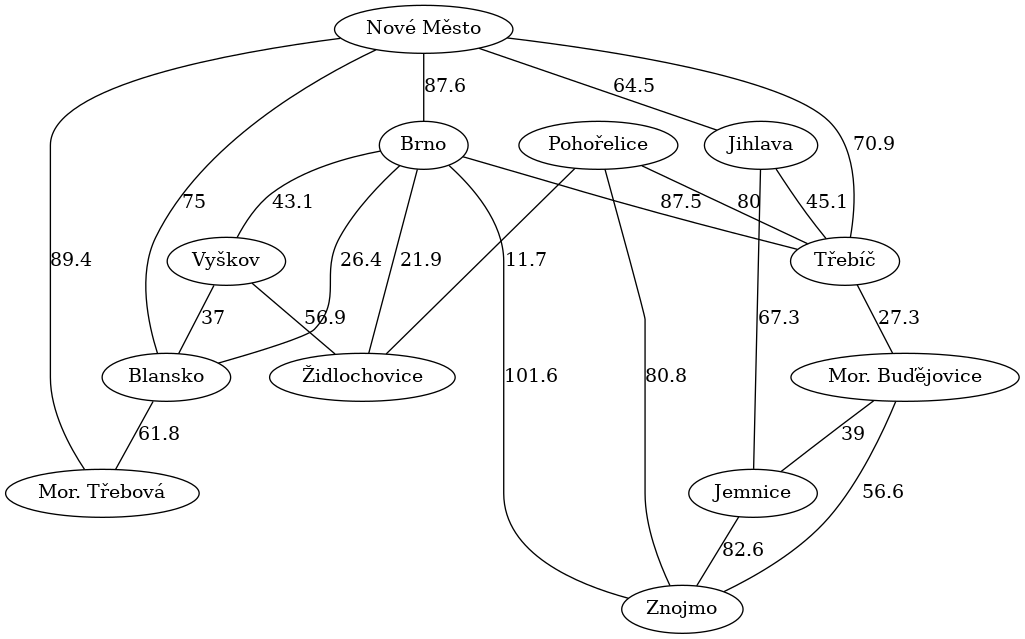Lets convert this graph to the new proposed implementation.

In :
use petgraph_evcxr::draw_dot;
use std::fmt::{self, Display, Write};
use petgraph::graph::*;

fn graph_to_timgraph<N, E, Ty, Ix>(g: &Graph<N, E, Ty, Ix>) -> TimGraph<N, E, Ty>
where
Ty: EdgeType,
Ix: IndexType,
E: Clone,
N: Clone,
{
let mut tg: TimGraph<N, E, Ty> = TimGraph {
nodes: Vec::with_capacity(g.node_count()),
ty: PhantomData,
};
for nodei in g.node_indices() {
tg.nodes.push(TimNode {
weight: g.node_weight(nodei).unwrap().clone(),
incomming: VecSet::new(),
outgoing: Vec::new(),
});
}
for edge in g.raw_edges().iter() {
tg.nodes[edge.target().index()].incomming.insert(edge.source().index());
tg.nodes[edge.source().index()].outgoing.push((edge.target().index(), edge.weight.clone()));
}
tg
}

fn draw_timgraph<N, E, Ty>(g: &TimGraph<N, E, Ty>)
where
Ty: EdgeType,
N: Display,
E: Display + Copy,
{
let mut dot = String::new();
let mut edges = String::new();
if Ty::is_directed() {
dot.push_str("digraph");
} else {
dot.push_str("graph");
}
dot.push_str("{\n");
for (i, node) in g.nodes.iter().enumerate() {
dot.push_str(&format!("\t{} [label=\"{}\"]\n", i, node.weight)); //TODO escape labels
for edge in node.outgoing.iter() {
let dir;
if Ty::is_directed() {
dir = "->";
} else {
dir = "--";
}
edges.push_str(&format!("\t{} {} {} [label=\"{}\"]\n", i, dir, edge.0, edge.1));
}
}
dot.push_str(&edges);
dot.push_str("}");
draw_dot(dot);
}

let tim_moravia = graph_to_timgraph(&moravia);

draw_timgraph(&tim_moravia);

Out: